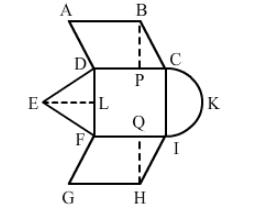# A child draws the figure of an aeroplane as shown.`
Question:

A child draws the figure of an aeroplane as shown. Here, the wings ABCD and FGHI are parallelograms, the tail DEF is an isosceles triangle, the cockpit CKI is a semicircle and CDFI is a square. In the given figure, BP ⊥ CDHQ ⊥ FI and EL ⊥ DF. If CD = 8 cm, BP = HQ = 4 cm and DE = EF = 5 cm, find the area of the whole figure.Solution:

CD = 8 cm
BP = HQ = 4 cm
DE = EF = 5 cm

Area of the parallelogram $\mathrm{ABCD}=B \times H$

$=8 \times 4$

$=32$ sq. $\mathrm{cm}$

Area of parallelogram $\mathrm{FGHI}=B \times H$

$=8 \times 4$

$=32$ sq. $\mathrm{cm}$

Area of the square $=\operatorname{Side}^{2}$

$=8^{2}=64$ sq. $\mathrm{cm}$

In $\Delta \mathrm{ELF}$, we have:

$\mathrm{EL}^{2}=5^{2}-4^{2}$

$\mathrm{EL}^{2}=9$

$\mathrm{EL}=3 \mathrm{~cm}$

Area of $\triangle \mathrm{DEF}=\frac{1}{2} \times B \times H$

$=\frac{1}{2} \times 8 \times 3$

$=12$ sq. $\mathrm{cm}$

Area of the semicircle $=\frac{1}{2} \pi r^{2}$

$=\frac{1}{2} \times \frac{22}{7} \times 16$

$=25.12$ sq. $\mathrm{cm}$

∴ Total Area =  Area of the parallelogram ABCD + Area of the parallelogram FGHI + Area of the triangle DEF + Area of the semicircle CKI + Area of the square
Total Area = 165.12 cm2